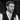# What is Memoization?

By Nick Scialli on February 13, 2020 | 🚀🚀 6 minute read

If you're enjoying this blog, please consider one or both of the following:

One type of programming concept I really like explaining is the type that has an intimidating name but, once you learn it, is actually a pretty simple concept. This is how I feel about memoization.

Not memorization—memoization. Let’s first see how Wikipedia describes memoization:

In computing, memoization or memoisation is an optimization technique used primarily to speed up computer programs by storing the results of expensive function calls and returning the cached result when the same inputs occur again.

I usually find Wikipedia descriptions to be dense and a bit unhelpful at first blush, but I actually find this one to be super helpful!

# The Basic Idea

Let’s say you have a function that takes some inputs, does something computationally expensive, and then returns an output. You call this function once, which is expensive. If you call the function again with the same exact inputs, why do the expensive computations again? Just use a cached result instead!

# An Simple Example Using JavaScript

Let’s create a super inefficient function to square a number in JavaScript. We’ll call it `inefficientSquare`:

``````function inefficientSquare(num) {
let result = 0;
for (let i = 0; i < num; i++) {
for (let j = 0; j < num; j++) {
result++;
}
}
return result;
}``````

This will square a number, but will do so super inefficiently. Let’s square the number 10,000 using this function and time how long it takes using the node performance timing API.

``````const { performance } = require('perf_hooks');
const start = performance.now();
inefficientSquare(10000);
const finish = performance.now();
console.log(finish - start);
// 115.8375``````

So this took me about 115 ms to run, but could be longer or shorter depending on your environment. Importantly, this is a pretty expensive function to run.

## Memoize It!

We know we’ll get the same result every time we square a number. Therefore, it’s a good candidate for memoization. If we calculate the square of a number once, why should we have to do that expensive calculation again? Let’s just save the result of that calculation if we ever need it.

Let’s create a `memoizedSquare` function that returns an function. This time, we’ll keep track of results that we’ve already seen.

``````function inefficientSquare(num) {
let result = 0;
for (let i = 0; i < num; i++) {
for (let j = 0; j < num; j++) {
result++;
}
}
return result;
}

function memoizedSquare() {
const cache = {};
return function(num) {
if (cache[num] === undefined) {
cache[num] = inefficientSquare(num);
}
return cache[num];
};
}``````

Now, we can see how our performance improves (dramatically) when we call the memoized function with the same value multiple times.

``````const squareFn = memoizedSquare();

// Call the first time: ineffience
const start = performance.now();
squareFn(10000);
const finish = performance.now();
console.log(finish - start);
// 117.0957

// Call the second time: speedy!
const start2 = performance.now();
squareFn(10000);
const finish2 = performance.now();
console.log(finish2 - start2);
// 0.005``````

And this makes sense—the second time we call `squareFn` with the parameter `10000`, we just retrieve the value from our `cache` object rather than doing the computation again.

This is memoization!

## Making a General Memoizer

Let’s make a general memoizer that will work for other functions as well.

``````function memoizer(fn) {
const cache = {};
return function(...args) {
const key = JSON.stringify(args);
if (cache[key] === undefined) {
cache[key] = fn(...args);
}
return cache[key];
};
}``````

We can demonstrate that this generalized memoizer works by applying it to our `inefficientSquare` function:

``````function inefficientSquare(num) {
let result = 0;
for (let i = 0; i < num; i++) {
for (let j = 0; j < num; j++) {
result++;
}
}
return result;
}

const squareFn = memoizer(inefficientSquare);

const start = performance.now();
squareFn(10000);
const finish = performance.now();
console.log(finish - start);
// 121.0662

const start2 = performance.now();
squareFn(10000);
const finish2 = performance.now();
console.log(finish2 - start2);
// 0.010``````

Great! We now have a `memoizer` function that takes another function as an argument and memoizes its results.

## Limitations of this Generalized Memoizer

The big limitation of this generalized memoizer is that we’re using `JSON.stringify` to turn our arguments into an object key. `JSON.stringify` is fairly limited: it can only stringify certain primitive and object types, so if we have arguments that can’t be stringified, this simple approach won’t be sufficient!

If you need a memoizer in production, I recommend using a well-vetted library like fast-memoize that uses other more complex memoization strategies for data types that can’t be easily stringified.

## A Note on Function Purity

To use memoization, your function should be pure! If you’re not famliar with the concept of pure functions, they essentially have two constaints: pure functions should have the same output given the same input, and pure functions shouldn’t have side effects.

# Conclusion

Memoization may sound like an intimidating concept, but really all it amounts to storing the result of an expensive computation to use later if necessary.

Happy coding!

References

[memoization on wikipedia](https://en.wikipedia.org/wiki/Memoization)Nick Scialli is a software engineer at the U.S. Digital Service.

### Subscribe to the mailing list!

If you like what I post here, please sign up to get updates and code insights in your inbox. I won't spam you and you can unsubscribe any time!